# Insulated Gate Field-Effect Transistors

## Discrete Semiconductor Devices and Circuits

• #### Question 1

 Don’t just sit there! Build something!!

Learning to mathematically analyze circuits requires much study and practice. Typically, students practice by working through lots of sample problems and checking their answers against those provided by the textbook or the instructor. While this is good, there is a much better way.

You will learn much more by actually building and analyzing real circuits, letting your test equipment provide the “answers” instead of a book or another person. For successful circuit-building exercises, follow these steps:

1. Carefully measure and record all component values prior to circuit construction, choosing resistor values high enough to make damage to any active components unlikely.
2. Draw the schematic diagram for the circuit to be analyzed.
3. Carefully build this circuit on a breadboard or other convenient medium.
4. Check the accuracy of the circuit’s construction, following each wire to each connection point, and verifying these elements one-by-one on the diagram.
5. Mathematically analyze the circuit, solving for all voltage and current values.
6. Carefully measure all voltages and currents, to verify the accuracy of your analysis.
7. If there are any substantial errors (greater than a few percent), carefully check your circuit’s construction against the diagram, then carefully re-calculate the values and re-measure.

When students are first learning about semiconductor devices, and are most likely to damage them by making improper connections in their circuits, I recommend they experiment with large, high-wattage components (1N4001 rectifying diodes, TO-220 or TO-3 case power transistors, etc.), and using dry-cell battery power sources rather than a benchtop power supply. This decreases the likelihood of component damage.

As usual, avoid very high and very low resistor values, to avoid measurement errors caused by meter “loading” (on the high end) and to avoid transistor burnout (on the low end). I recommend resistors between 1 kΩ and 100 kΩ.

One way you can save time and reduce the possibility of error is to begin with a very simple circuit and incrementally add components to increase its complexity after each analysis, rather than building a whole new circuit for each practice problem. Another time-saving technique is to re-use the same components in a variety of different circuit configurations. This way, you won’t have to measure any component’s value more than once.

• #### Question 2

The following illustration is a cross-section of an insulated gate field-effect transistor, sometimes referred to as an IGFET: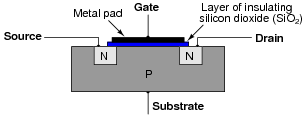Explain what happens when a positive voltage is applied to the gate (with reference to the substrate), with regard to electrical conductivity between the source and drain: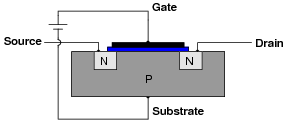• #### Question 3

The letters “MOS” in the acronym “MOSFET” stand for “Metal Oxide Semiconductor”. Describe what this means, in reference to the construction of a MOSFET.

• #### Question 4

Some types of MOSFETs have a source-drain channel already formed with no applied gate voltage: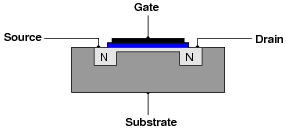Explain what happens to source-drain conductivity with each of the following applied gate-to-substrate voltages. Modify the illustrations if necessary: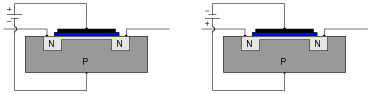• #### Question 5

There are two general classes of MOSFETs: MOSFETs that conduct with no applied gate voltage, and MOSFETs that require a gate voltage to be applied for conduction. What are each of these MOSFET types called, and what are their respective schematic symbols?

Each of the symbols for these different types of MOSFETs hold clues to the transistor types they represent. Explain how the symbols hint at the characteristics of their respective transistor types.

• #### Question 6

Identify these schematic symbols:• #### Question 7

Field effect transistors are classified as majority carrier devices. Explain why.

• #### Question 8

Bipolar junction transistors (BJTs) are considered “normally-off” devices, because their natural state with no signal applied to the base is no conduction between emitter and collector, like an open switch. Are insulate-gate field-effect transistors (IGFETs) considered the same? Why or why not?

• #### Question 9

The typical amount of current through a MOSFET gate terminal is far less than the typical amount of current through a BJT base terminal, for similar controlled currents (drain or collector, respectively). Explain what it is about the construction and/or use of the MOSFET that limits the input current to almost nothing during normal operation.

• #### Question 10

Metal Oxide Field-Effect Transistors (MOSFETs) differ in behavior from Bipolar Junction Transistors (BJTs) in several ways. Address each one of these behavioral aspects in your answer:

Current gain
Conduction with no input (gate/base) signal
Polarization
• #### Question 11

E-type MOSFETs are normally-off devices just like bipolar junction transistors, the natural state of their channels strongly resisting the passage of electric currents. Thus, a state of conduction will only occur on command from an external source.

Explain what must be done to an E-type MOSFET, specifically, to drive it into a state of conduction (where a channel forms to conduct current between source and drain).

• #### Question 12

D-type MOSFETs are normally-on devices just like junction field-effect transistors, the natural state of their channels being passable to electric currents. Thus, a state of cutoff will only occur on command from an external source.

Explain what must be done to an D-type MOSFET, specifically, to drive it into a state of cutoff (where the channel is fully depleted).

• #### Question 13

Metal Oxide Field-Effect Transistors (MOSFETs) differ in some regards from Junction Field-Effect Transistors (JFETs). Explain in your own words what the difference(s) is/are.

• #### Question 14

Identify each type of MOSFET (whether it is N-channel or P-channel, D-type or E-type), label the terminals, and determine whether the MOSFET in each of these circuits will be turned on or off:• #### Question 15

Identify each type of MOSFET (whether it is N-channel or P-channel, D-type or E-type), label the terminals, and determine whether the MOSFET in each of these circuits will be turned on or off: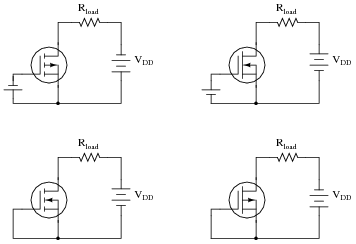• #### Question 16

Identify each type of MOSFET (whether it is N-channel or P-channel, D-type or E-type), label the terminals, and determine whether the MOSFET in each of these circuits will be turned on or off:• #### Question 17

Explain why a circuit designer would choose a MOSFET over a bipolar transistor for a certain application. What advantage(s) does a MOSFET have over a bipolar transistor?

Challenge question: prove your point by comparing parametric ratings from two transistor datasheets, one bipolar and the other an insulated-gate field effect. Be sure these two transistors have similar controlled current ratings (maximum collector current and drain current, respectively).

• #### Question 18

What does the term transconductance mean, with reference to a field-effect transistor? Is the transconductance function for an FET a linear or a nonlinear relationship? Explain why, making reference to an equation if at all possible to explain your answer.

• #### Question 19

The “substrate” connection in a MOSFET is often internally connected to the source, like this: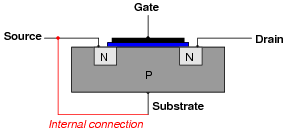This turns the MOSFET from a four-terminal device into a three-terminal device, making it easier to use. One consequence of this internal connection, though, is the creation of a parasitic diode between the source and drain terminals: a PN junction that exists whether we want it to or not.

Add this parasitic diode to the MOSFET symbol shown here (representing the MOSFET cross-section shown above), and explain how its presence affects the transistor’s use in a real circuit: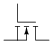• #### Question 20

A technician is using a digital multimeter (with a “diode check” feature) to identify the terminals of a power MOSFET: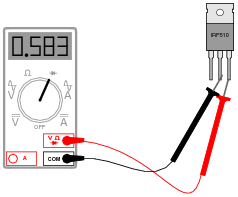The technician obtains the following “diode check” voltage measurements, in this order:

1. Black lead on middle terminal, Red lead on right terminal = 0.583 volts (shown in illustration)
2. Red lead on middle terminal, Black lead on right terminal = O.L. (open)
3. Black lead on middle terminal, Red lead on left terminal = O.L. (open)
4. Black lead on middle terminal, Red lead on right terminal = 0.001 volts
5. Red lead on middle terminal, Black lead on right terminal = 0.001 volts

Explain why the fourth and fifth measurements are so different from the first and second, respectively, when they were taken between the same terminals on the MOSFET. Hint: this particular MOSFET is an N-channel, enhancement-type.

• #### Question 21

An important consideration when working around circuits containing MOSFETs is electrostatic discharge, or ESD. Describe what this phenomenon is, and why it is an important consideration for MOSFET circuits.

• #### Question 22

Anti-static wrist straps are commonly worn by technicians when performing work on circuits containing MOSFETs. Explain how these straps are used, and how you would test one to ensure it is functioning properly.

• #### Question 23

Complete the circuit, showing how the pushbutton switch could be connected to the gate of the MOSFET in order to exert control over the load:• #### Question 24

Determine whether the load is energized or de-energized with the switch in the position shown. Also, identify whether the transistor is a depletion type or an enhancement type:• #### Question 25

Determine whether the load is energized or de-energized with the switch in the position shown. Also, identify whether the transistor is a depletion type or an enhancement type:• #### Question 26

Determine whether the load is energized or de-energized with the switch in the position shown. Also, identify whether the transistor is a depletion type or an enhancement type:• #### Question 27

Determine whether the load is energized or de-energized with the switch in the position shown. Also, identify whether the transistor is a depletion type or an enhancement type: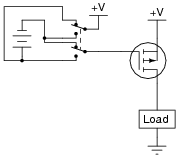• #### Question 28

Determine whether the load is energized or de-energized with the switch in the position shown. Also, identify whether the transistor is a depletion type or an enhancement type:• #### Question 29

It is often necessary to have a power transistor source current to a load (provide a path from the positive supply voltage rail to the load) rather than sink current from the load (provide a path from the load to the negative voltage rail or ground), because one side of the load is already connected to ground: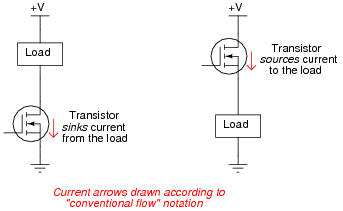When the transistor sources current, it is often referred to as a high-side switch. Determine the driving voltage requirements for each of these high-side MOSFET switches; that is, determine what must be connected to the gate of each transistor to fully turn it on so that the load receives full power:• #### Question 30

Draw the proper wire connections necessary for “enhancing” this MOSFET with the solar cell’s voltage, so that the battery energizes the relay whenever there is sufficient light exposure on the solar cell:• #### Question 31

Explain what will happen in this circuit when each pushbutton switch is individually actuated:Can you think of any practical applications for a circuit like this?

• #### Question 32

This circuit uses a combination of capacitance and resistance to produce a time delay when the pushbutton switch is released, causing the lamp to remain on for a short while after the switch opens: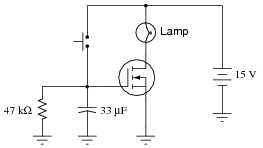Calculate how long the lamp will remain on after the switch opens, assuming the MOSFET has a gate threshold (turn-on) voltage of VGS(th) = 4 volts.

• #### Question 33

Predict how this circuit will be affected as a result of the following faults. Consider each fault independently (i.e. one at a time, no multiple faults):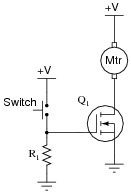Transistor Q1 fails open (drain-to-source):
Transistor Q1 fails shorted (drain-to-source):
Resistor R1 fails open:

For each of these conditions, explain why the resulting effects will occur.

• #### Question 34

Predict how this circuit will be affected as a result of the following faults. Consider each fault independently (i.e. one at a time, no multiple faults):Transistor Q1 fails shorted (collector-to-emitter):
Transistor Q2 fails open (drain-to-source):
Resistor R1 fails open:
Resistor R2 fails open:

For each of these conditions, explain why the resulting effects will occur.

• #### Question 35

Predict how this circuit will be affected as a result of the following faults. Consider each fault independently (i.e. one at a time, no multiple faults):Transistor Q1 fails shorted (drain-to-source):
Transistor Q2 fails shorted (drain-to-source):
Resistor R1 fails open:
Resistor R2 fails open:
Solder bridge (short) past resistor R1:

For each of these conditions, explain why the resulting effects will occur.

• #### Question 36

A very useful MOSFET circuit is the bilateral switch, an example shown here for you to analyze:The “dual inverter” circuit simply ensures the two control lines A and B will always be opposite polarities (one at Vdd potential, the other at ground potential).

What is the purpose of a “bilateral switch” circuit? Hint: there are two integrated circuit implementations of the bilateral switch - the 4016 and the 4066. Investigate the datasheets for these integrated circuits to learn more!

• #### Question 37

A special type of insulated-gate field-effect transistor is the dual gate MOSFET, shown here: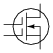Draw a schematic diagram using normal (single-gate) MOSFETs, equivalent to this dual-gate MOSFET.

• #### Question 38

A potential problem for power MOSFETs is $$\frac{dv}{dt}$$ induced turn-on. Explain why a MOSFET may turn on when it’s not supposed to, given an excessive $$\frac{dv}{dt}$$ condition.

• #### Question 39

Find one or two real insulated-gate field-effect transistors and bring them with you to class for discussion. Identify as much information as you can about your transistors prior to discussion:

Terminal identification (which terminal is gate, source, drain)
Continuous power rating
Typical transconductance

Note: be careful to keep your transistors in anti-static foam as much as possible, to avoid damage to the gate from electrostatic discharge.